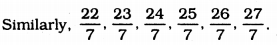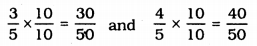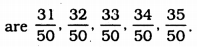# KSEEB Solutions for Class 9 Maths Chapter 1 Number Systems Ex 1.1

KSEEB Solutions for Class 9 Maths Chapter 1 Number Systems Ex 1.1 are part of KSEEB Solutions for Class 9 Maths. Here we have given Karnataka Board Class 9 Maths Chapter 1 Number Systems Exercise 1.1.

## Karnataka Board Class 9 Maths Chapter 1 Number Systems Ex 1.1

KSEEB Solutions For Class 9 Maths Question 1.
Is a zero a rational number? Can you write it in the form $$\frac{p}{q}$$, where p and q are integers and q ≠ 0 ? (p, q,ϵ Z, q ≠ 0)
Zero is a rational number.
This can be written in the form of $$\frac{p}{q}$$ because $$\frac{o}{q}$$ is a rational number.
E.g. $$\frac{0}{2}=0, \quad \frac{0}{5}=0$$. etc.
Zero belongs to set of rational number.

KSEEB Solutions For Class 9 Maths Number System Question 2.
Find six rational numbers between 3 and 4.
We can write six rational numbers between 3 and 4 as
$$3=\frac{21}{7} \text { and } 4=\frac{28}{7}$$
∴ rational numbers between $$\frac{21}{7}$$ and $$\frac{28}{7}$$.KSEEB Solutions For Class 9 Maths Chapter 1 Question 3.
Find five rational numbers between $$\frac{3}{5}$$ and $$\frac{4}{5}$$
Rational numbers between $$\frac{3}{5}$$ and $$\frac{4}{5}$$ are∴ Rational numbers between $$\frac{30}{50}$$ and $$\frac{40}{50}$$KSEEB Solutions For Class 9 Maths Chapter 1 Number System Question 4.
State whether the following statements are true or false. Give reasons for your answers :
(i) Every natural number is a whole number.
False. Because $$\frac{1}{2}$$ is a rational number but not a whole number.